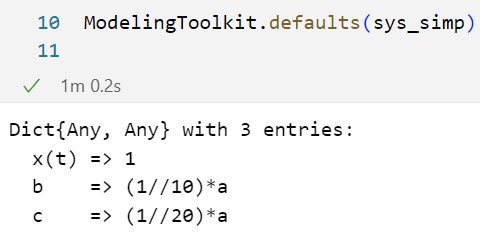# MTK & missing parameters, MWE

OK – I’m trying again – with a MWE. Consider this an observation (I’m not saying this should be changed in MTK). This post is more meant as a request for advice on best practice.

Consider the DAE

\frac{dx}{dt} = -b\cdot (x-z) \\ 0 = z - c\cdot x

where b=a/10 and c=a/20, and I want to study uncertainty in a.

Suppose I do the following:

using ModelingToolkit

@parameters a=10 b=a/10 c=a/20

@variables t
Dt = Differential(t)

@variables x(t)=1 z(t)

eqs = [Dt(x) ~ - b*(x-z),
0 ~ z - c*x]

@named sys = ODESystem(eqs)

sys_simp = structural_simplify(sys)

ModelingToolkit.defaults(sys_simp)Observe that the default value a=10 seems to have disappeared. This is confirmed – kind of – when I try to make a numeric version of the model:

> tspan = (0.0, 10)
> prob = ODEProblem(sys_simp,[],tspan)

Output exceeds the size limit. Open the full output data in a text editorMethodError: no method matching AbstractFloat(::Type{SymbolicUtils.BasicSymbolic{Real}})
Closest candidates are:
(::Type{T})(!Matched::AbstractChar) where T<:Union{AbstractChar,


Suspicion: My guess is that MTK has stripped off all parametes in sys/sys_simp that are not used explicitly in the equations. Thus, information about the value of a has disappeared.

OK – because I only have uncertainty in the single parameter a, and not independently in b and c, I cannot really drop a and replace the parameters by b=1 and c=1/2.

So what is the “correct” way to get around this? I have tried to define a as a constant instead, in the hope that the constant will be carried over to the system sys/sys_simp. But no dice – seems like also constants must appear explicitly to be included in the system (sys/sys_simp).

Possible solution: keep a as a parameter, and instead specify b and c as variables:

using ModelingToolkit
using DifferentialEquations

@parameters a=10.0
@variables t
Dt = Differential(t)
@variables x(t)=1.0 z(t) b(t) c(t)

eqs = [Dt(x) ~ - b*(x-z),
0 ~ z - c*x,
b ~ a/10,
c ~ a/20]

@named sys = ODESystem(eqs)

sys_simp = structural_simplify(sys)
tspan = (0.0,10)

prob = ODEProblem(sys_simp,[],tspan)

sol = solve(prob)

sol(5, idxs=x)
sol(5, idxs=z)
sol(5, idxs=b)
sol(5, idxs=c)


With this strategy, the model runs and produces sensible results.

QUESTION: is this strategy of making parameters into variables the recommended strategy?

When using ODESystem with 1 input (eqs), I think MTK might struggle to determine the full system information. I recommend using the 4 input version:

using ModelingToolkit

pars = @parameters a=10 b=a/10 c=a/20

@variables t
Dt = Differential(t)

vars = @variables x(t)=1 z(t)

eqs = [Dt(x) ~ - b*(x-z),
0 ~ z - c*x]

@named sys = ODESystem(eqs, t, vars, pars)

sys_simp = structural_simplify(sys)

ModelingToolkit.defaults(sys_simp)


Which keeps a in the defaults

Dict{Any, Any} with 4 entries:
a    => 10
x(t) => 1
b    => (1//10)*a
c    => (1//20)*a

1 Like

Thanks for clarification! Hm… where do I find information about the “1 input” vs. “4 input” version? I looked up the info on constructor ODESystem in the documentation, and found the following (snippet):

Very useful to find info on fields. However, I don’t find info on constructor arguments… except for the following at the end of the fields list:

Here, a 5 input version is listed as an example.

• But is there a 6 input version, too?
• Should I use methods(ODESystem) to find all possible args (and their order) and kwargs?
• How do I know what is default? Do I need to know this?
• Or is this detailed in the documentation?

If you run methods(ODESystem) you can see there are actually 12 ways to construct an ODESystem. What you refer to a 5 input version is actually 4 inputs with 1 keyword argument tspan which is the first method listed…

julia> methods(ODESystem)
# 12 methods for type constructor:
 ODESystem(deqs::AbstractVector{<:Equation}, iv, dvs, ps; controls, observed, systems, tspan, name, default_u0, default_p, defaults, connector_type, preface, continuous_events, discrete_events, checks, metadata, gui_metadata)